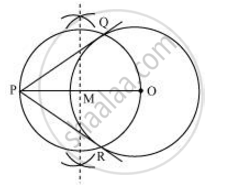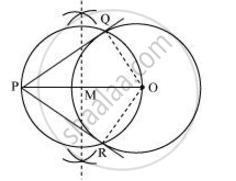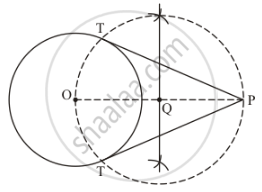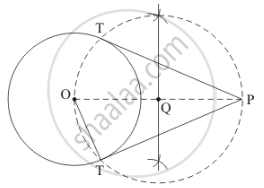Share
Notifications

View all notifications
Books Shortlist
Your shortlist is empty

# Draw a circle of radius 6 cm. From a point 10 cm away from its centre, construct the pair of tangents to the circle and measure their lengths. Give the justification of the construction. - CBSE Class 10 - Mathematics

Login
Create free account

Forgot password?
ConceptConstruction of Tangents to a Circle

#### Question

Draw a circle of radius 6 cm. From a point 10 cm away from its centre, construct the pair of tangents to the circle and measure their lengths. Give the justification of the construction.

#### Solution 1

A pair of tangents to the given circle can be constructed as follows.

Step 1

Taking any point O of the given plane as centre, draw a circle of 6 cm radius. Locate a point P, 10 cm away from O. Join OP.

Step 2

Bisect OP. Let M be the mid-point of PO.

Step 3

Taking M as centre and MO as radius, draw a circle.

Step 4

Let this circle intersect the previous circle at point Q and R.

Step 5

Join PQ and PR. PQ and PR are the required tangents.The lengths of tangents PQ and PR are 8 cm each.

Justification

The construction can be justified by proving that PQ and PR are the tangents to the circle (whose centre is O and radius is 6 cm). For this, join OQ and OR.∠PQO is an angle in the semi-circle. We know that angle in a semi-circle is a right angle.

∴ ∠PQO = 90°

⇒ OQ ⊥ PQ

Since OQ is the radius of the circle, PQ has to be a tangent of the circle. Similarly, PR is a tangent of the circle.

#### Solution 2

Given that

Construct a circle of radius 6 cm, and let a point P = 10 cm form its centre, construct the pair of tangents to the circle.

Find the length of tangents.

We follow the following steps to construct the givenStep of construction

Step: I- First of all we draw a circle of radius AB = 6 cm.

Step: II- Make a point P at a distance of OP = 10 cm, and join OP.

Step: III -Draw a right bisector of OP, intersecting OP at Q .

Step: IV- Taking as centre and radius OQ = PQ, draw a circle to intersect the given circle at T and T’.

Step: V- Joins PT and PT’ to obtain the require tangents.

Thus, PT and P'T' are the required tangents.

Find the length of tangents.As we know that OT ⊥ PT and ΔOPT is right triangle.

Therefore,

OT = 6cm and PO = 10cm

In ΔOPT,

PT2 = OP2 - OT2

PT2 = 102 - 62

PT2 = 100 - 36

PT2 = 64

PT = sqrt64=8

Thus, the length of tangents = 8 cm.

Is there an error in this question or solution?

#### APPEARS IN

NCERT Solution for Mathematics Textbook for Class 10 (2019 to Current)
Chapter 11: Constructions
Ex.11.20 | Q: 1 | Page no. 221
NCERT Solution for Mathematics Textbook for Class 10 (2018 to Current)
Chapter 11: Constructions
Ex.11.20 | Q: 1 | Page no. 221
Solution Draw a circle of radius 6 cm. From a point 10 cm away from its centre, construct the pair of tangents to the circle and measure their lengths. Give the justification of the construction. Concept: Construction of Tangents to a Circle.
S